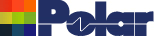Calculation of controlled impedance traces by 2D Field solving Application Note AP135 Calculation of controlled impedance traces by 2D Field solving using Green's function and method of moments Controlled/characteristic impedance The controlled or characteristic impedance of a pair of conductors on a printed circuit board, is the ratio of voltage to current of the propagating pulse or step voltage. For the pulse or step to remain unchanged as it propagates, the impedance must be the same at each point on the propagation path. Reflections occur when the pulse encounters an impedance different from the controlled impedance. For a single track above a ground plane, the controlled impedance is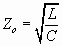(1.1) where L is the inductance and C is the capacitance per unit length of the track. A pair of tracks of the same cross-section (i.e. balanced), above a ground plane, have an impedance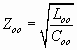(1.2) to ground when they are excited by an equal and opposite voltage to the ground voltage. Zoo is also known as the odd mode impedance.  Loo and  Coo are, respectively, the odd mode inductance and capacitance per unit length. The impedance between the tracks is the differential impedance,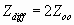(1.3) It is only possible to derive algebraic equations for the various inductances and capacitances for a very limited number of track configurations, usually when the track thickness is zero. Modern tracks may be relatively thick, and not rectangular, because of the effect of the etching process. In these circumstances, the value of the inductance and capacitance, and hence impedance, must be obtained directly from electromagnetic field calculations. For striplines which have a uniform substrate, dielectric constant er can be written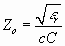(1.4) where c is the velocity of light. For both surface and embedded microstrip lines, (1.1), can be written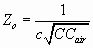(1.5) where Cair is the capacitance of the same configuration with air as the substrate. The effective dielectric constant is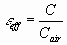(1.6) Similar equations for Zoo also apply. Calculation of Capacitance The equations, in section 1, assume that the electric fields can be obtained from electrostatic field theory. This is acceptable when the track dimensions are small relative to the wavelength of the highest frequency component in a pulse or step. When the track is at voltage V, relative to the ground, then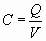(2.1) where Q is the charge per unit length of track. In (2.1) , C can be any of the capacitances in the equations of section 1. The charge density, q over the track cross-section, is not uniform: there will be a much greater density at track corners and edges. At the pulse rates and frequencies involved, the charge density resides on the track surface. If the distribution of q is known, then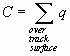(2.2) Many methods are available to estimate q. A popular method is the finite element method. In this method, the whole of the transverse plane containing the track is divided into a mesh of triangles of different sizes. The relation between the unknown voltages at the nodes of the triangles is based on the minimum electrostatic energy and is known. Some nodes are on the surface of the track. At these nodes the voltage is known. Then by solving a matrix equation, all the unknown voltages and track surface charge densities are found. The difficulty with this method is that the voltages in the space around the track are unnecessarily calculated. Also it is difficult to ensure that the high charge density at the track corners is adequately modelled since most field solvers using this method make several passes through the program each time with a finer mesh. The mesh sizes are determined within the software. The program terminates when there is very little change between adjacent passes. The method used in CITS25 and SI6000 to estimate q is the Method of Moments. This method is based on the direct relation between voltage and charge in an electrostatic field. The voltage V at a distance r from a charge q in a two dimensional region is: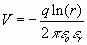(2.3) where eo is the absolute permittivity and er is the dielectric constant of the region. (2.3) can be written as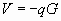(2.4) where G is known as the Green's1 function. For a simple two dimensional region, G can be found by comparing (2.4) with (2.3). When q is a continuous distribution of charge, then is modified to read: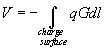(2.5) To apply the method of moments, the track is assumed to have a known voltage, V. The track surface is assigned nodes which have a predetermined very non-linear distribution. These nodes are very close together at the corners. An unknown charge, qj, is assigned to the nodes. The charge distribution between nodes is assumed to be linear. Using (2.5) with q replaced by qj, the known voltage, Vi at the nodes, is calculated from the charge at all the nodes. This is known as point matching.The integration in (2.5) is obtained numerically using Gauss integration. This process leads to the matrix equation(2.6) where A is a known square matrix derived from the application of (2.5) with the appropriate Green’s function, q is a vector of the unknown nodal charges and Vis a vector of the known nodal voltages. The solution of (2.6) yields the nodal charges. Using the nodal charges, the track charge Q is obtained by summation assuming the linear charge distribution between nodes, from which the capacitance and impedance is calculated. In the microstrip case, there are two calculations required; the first with the substrate, and the second with the substrate replaced by air. The above is an outline of the calculation. The calculation uses the appropriate Green’s function for the track configuration. The steps used to obtain the Green’s function for the track configurations, is described in the next section The Green’s Functions A simple two dimensional Green’s function is outlined in (2.4). However this value cannot be used directly in the calculation, because there are ground planes and substrate surfaces present. In a stripline there are two ground planes. A charge q lies between them in a uniform substrate. Fig 1 shows the general arrangement.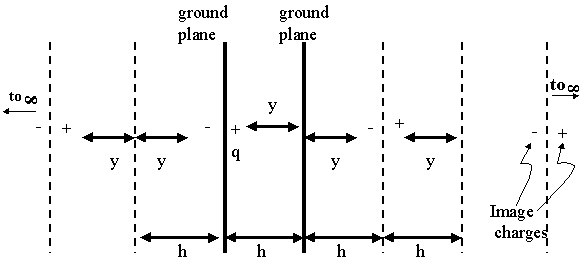Fig. 1. Charge between Ground Planes The charge images are of equal and opposite sign and extend to infinity in each direction. The ground planes can be notionally removed, and the charge images used instead. The Green’s function is obtained by summing, algebraically, the individual effect of an infinite number of charges, with q equal to 1. Fig. 2 shows the images for a charge q above the substrate of a surface microstrip.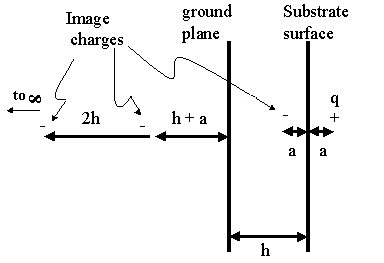Fig. 2. Charge above Substrate Surface There are image charges produced by the multiple reflections between the substrate surface and the ground plane. The images are of the opposite sign to that of q and decrease in amplitude as the distance is increased. The ground plane and substrate surface are replaced by the infinite number of image charges. The Green’s function is the sum of the effect of these images when q equals 1. The sum cannot be obtained analytically. Fortunately the infinite sum quickly converges as the number of terms increases. The sum is readily calculated numerically to high degree of accuracy. The Green’s function for embedded microstrip, is derived in a similar manner to that for surface microstrip, except that the pattern of images is similar to those for stripline. Calculating the impedance The program used to calculate the impedances, assumes that, in manufacture, the tracks are etched to a trapezoidal shape. For single tracks, the whole width of the track is used. For pairs of tracks, symmetry is assumed. Only nodes on one track need then be used. The odd mode impedance Zoo is first calculated. The differential impedance is then obtained using (1.3). Within the program, all dimensions are normalised to the substrate thickness; this means that any consistent set of dimensions can be used to define the track configuration. During the development of the software various combinations of the number of nodes and their non-linear distribution were examined. The impedances calculated were compared with the impedances for those few cases were there is an exact result. This comparison shows that the impedances calculated by Polar field solvers are accurate to at least 3 significant figures in the range 20 - 150Ohms . 1. From Mr George Green, the 19th Century English Mathematician who developed Green's function Dr J. Alan Staniforth Alan Staniforth received a PhD in 1959 from Sheffield University, UK. He was a full time lecturer from 1960 to 1992 and is now a part time lecturer at Salford University, UK, in the School of Acoustics and Electronics. He has published several articles on microwave transmission and on the electromagnetic transients in heavy particle accelerators. For many years he was a consultant to the Science and Engineering Research Council, UK. Dr Staniforth is now a consultant to Polar Instruments Ltd.